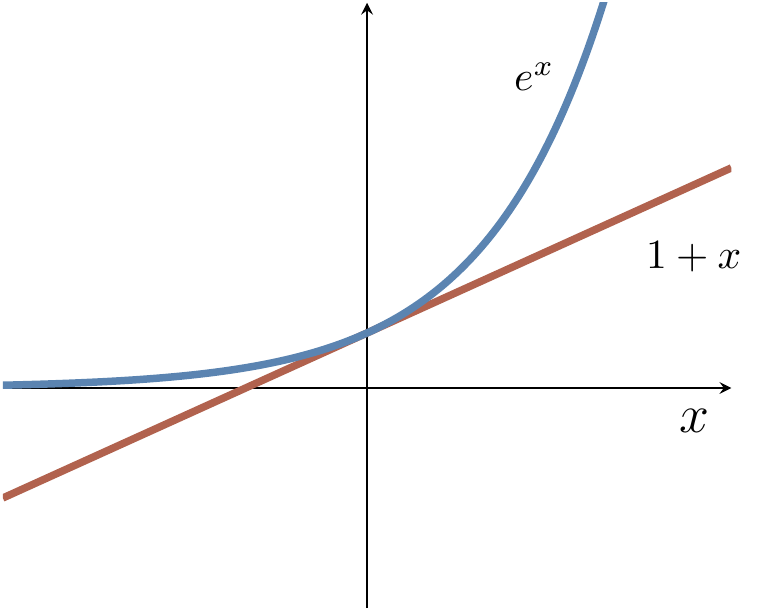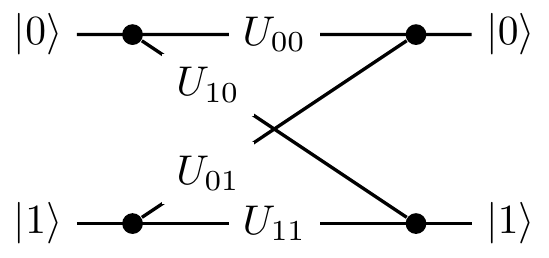## 2.2 Quantum bits, called “qubits”

Such a two-state machine that we have just described in abstract terms is usually realised as a controlled evolution of a two-state system, called a quantum bit, or qubit for short.47 For example, the state |0\rangle may be chosen to be the lowest energy state of an atom (the ground state), and state |1\rangle a higher energy state (the excited state). Pulses of light of the appropriate frequency, duration, and intensity can take the atom back and forth between the basis states |0\rangle and |1\rangle (implementing logical \texttt{NOT}).

Some other pulses (say, half the duration or intensity) will take the atom into states that have no classical analogue. Such states are called coherent superpositions of |0\rangle and |1\rangle, and represent a qubit in state |0\rangle with some amplitude \alpha_0 and in state |1\rangle with some other amplitude \alpha_1. This is conveniently represented by a state vector |\psi\rangle = \alpha_0|0\rangle + \alpha_1|1\rangle \leftrightarrow \begin{bmatrix} \alpha_0 \\\alpha_1 \end{bmatrix}By Born’s rule, we know that \alpha_0 and \alpha_1 cannot be arbitrary complex numbers: they must satisfy |\alpha_0|^2+|\alpha_1|^2=1. This lets us draw the state vector “geometrically”, using the fact that the locus of vectors of magnitude equal to 1 describes a circle:But recall that amplitudes are complex numbers, and so \alpha_0 and \alpha_1 cannot really be drawn as 1-dimensional real vectors on a flat screen or piece of paper; the picture above provides good intuition, but to be fully accurate we would need to draw it in four-dimensional space (or at least on some three-dimensional paper).

A qubit is a quantum system in which the Boolean states 0 and 1 are represented by a prescribed pair of normalised and mutually orthogonal quantum states labelled by |0\rangle and |1\rangle. The two states form a so-called computational (or standard) basis, and so any other state of an isolated qubit can be written as a coherent superposition |\psi\rangle = \alpha_0|0\rangle + \alpha_1|1\rangle for some \alpha_0 and \alpha_1 such that |\alpha_0|^2 + |\alpha_1|^2 = 1.

In practice, a qubit is typically a microscopic system, such as an atom, a nuclear spin, or a polarised photon.

As we have already mentioned, any48 computational step, that is, any physically admissible operation U on a qubit, is described by a (2\times 2) unitary matrix U. It modifies the state of the qubit as |\psi\rangle \longmapsto |\psi'\rangle = U|\psi\rangle which we can write explicitly as \begin{bmatrix} \alpha'_0 \\\alpha'_1 \end{bmatrix} = \begin{bmatrix} U_{00} & U_{01} \\U_{10} & U_{11} \end{bmatrix} \begin{bmatrix} \alpha_0 \\\alpha_1 \end{bmatrix} That is, the operation U turns the state |\psi\rangle, with components \alpha_k, into the state |\psi'\rangle=U|\psi\rangle, with components \alpha'_l= \sum_k U_{lk}\alpha_k.

1. More general n-state systems can also be of interest, and are sometimes called q-nits; three-state systems in particular are sometimes called qutrits. In this book, however, we will only concern ourselves with qubits, since they readily generalise the classical notion of bits (and also give us more than enough interesting constructions to get started with!).↩︎

2. Here we are talking about isolated systems. As you will soon learn, a larger class of physically admissible operations is described by completely positive maps. It may sound awfully complicated but, as you will soon see, it is actually very simple.↩︎